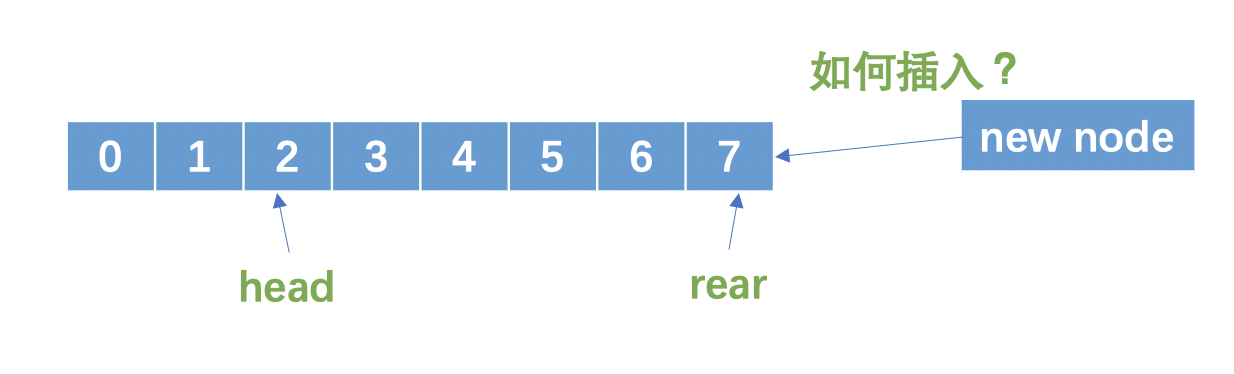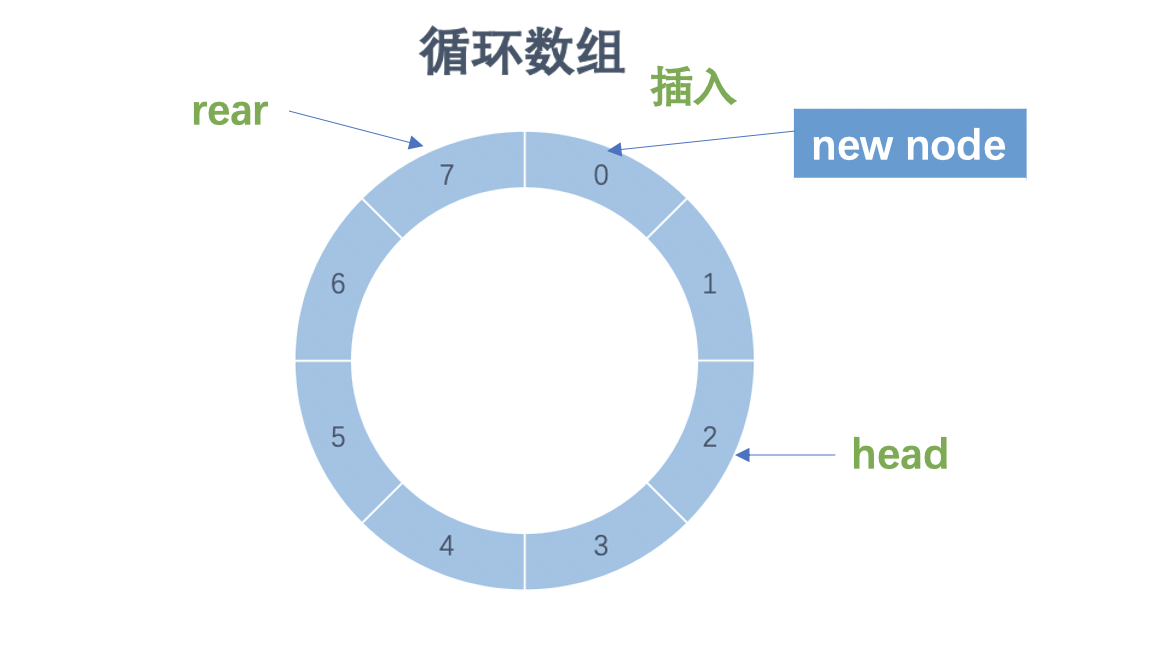看动画学算法之:队列queue

简介队列的实现队列的数组实现public class ArrayQueue {

//存储数据的数组
private int[] array;
//real索引
private int rear;
//数组容量
private int capacity;

public ArrayQueue (int capacity){
this.capacity=capacity;
this.rear =-1;
this.array= new int[capacity];
}

public boolean isEmpty(){
}

public boolean isFull(){
}

public int getQueueSize(){
return 0;
}
}

//从尾部入队列
public void enQueue(int data){
if(isFull()){
System.out.println("Queue is full");
}else{
//从尾部插入
rear = (rear +1)%capacity;
array[rear]= data;
}
}
}

//从头部取数据
public int deQueue(){
int data;
if(isEmpty()){
System.out.println("Queue is empty");
return -1;
}else{
rear = -1;
}else{
}
return data;
}
}
}

rear = (rear +1)%capacity

队列的动态数组实现//因为是循环数组，这里不能做简单的数组拷贝
private void extendQueue(){
int newCapacity= capacity*2;
int[] newArray= new int[newCapacity];
//先全部拷贝
System.arraycopy(array,0,newArray,0,array.length);
newArray[i]= -1;
//拷贝到新的位置
newArray[i+capacity]=array[i];
}
//重置real的位置
rear= rear+capacity;
//重置capacity和array
capacity=newCapacity;
array=newArray;
}
}

队列的链表实现//rear节点
private Node rearNode;

class Node {
int data;
Node next;
//Node的构造函数
Node(int d) {
data = d;
}
}

public boolean isEmpty(){
}

public void enQueue(int data){
Node newNode= new Node(data);
//将rearNode的next指向新插入的节点
if(rearNode !=null){
rearNode.next=newNode;
}
rearNode=newNode;
}
}

public int deQueue(){
int data;
if(isEmpty()){
System.out.println("Queue is empty");
return -1;
}else{
}
return data;
}
}

队列的时间复杂度learn-algorithm